﻿ Common Core Standards for Math - KindergartenAdaptive Worksheets

Helping Every Child to Succeed

toll-free (888) 777-0876
fax (888) 777-0875

## Number and Operations in Base Ten - Fractions

#### Use equivalent fractions as a strategy to add and subtract fractions.

5.NF.A.1

Add and subtract fractions with unlike denominators (including mixed numbers) by replacing given fractions with equivalent fractions in such a way as to produce an equivalent sum or difference of fractions with like denominators. For example, 2/3 + 5/4 = 8/12 + 15/12 = 23/12. (In general, a/b + c/d = (ad + bc)/bd.)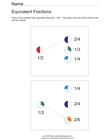Equivalent Fractions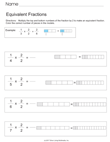Equivalent Fractions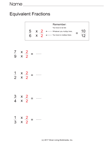Equivalent Fractions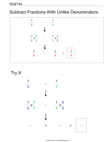Subtract Fractions with Different Denominators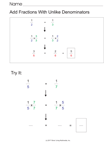Add Fractions with Different Denominators

5.NF.A.2
Solve word problems involving addition and subtraction of fractions referring to the same whole, including cases of unlike denominators, e.g., by using visual fraction models or equations to represent the problem. Use benchmark fractions and number sense of fractions to estimate mentally and assess the reasonableness of answers. For example, recognize an incorrect result 2/5 + 1/2 = 3/7, by observing that 3/7 < 1/2.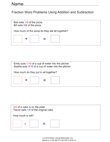Fraction Word Problems With Addition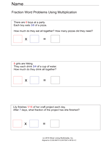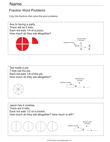Fraction Word Problems With Multiplication

#### Apply and extend previous understandings of multiplication and division.

5.NF.B.3
Interpret a fraction as division of the numerator by the denominator (a/b = a÷ b). Solve word problems involving division of whole numbers leading to answers in the form of fractions or mixed numbers, e.g., by using visual fraction models or equations to represent the problem. For example, interpret 3/4 as the result of dividing 3 by 4, noting that 3/4 multiplied by 4 equals 3, and that when 3 wholes are shared equally among 4 people each person has a share of size 3/4. If 9 people want to share a 50-pound sack of rice equally by weight, how many pounds of rice should each person get? Between what two whole numbers does your answer lie?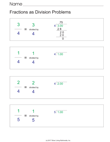Fractions As Division Problems

5.NF.B.4.
Apply and extend previous understandings of multiplication to multiply a fraction or whole number by a fraction.
5.NF.B.4.A
Interpret the product (a/b) × q as a parts of a partition of q into b equal parts; equivalently, as the result of a sequence of operations a × q ÷ b. For example, use a visual fraction model to show (2/3) × 4 = 8/3, and create a story context for this equation. Do the same with (2/3) × (4/5) = 8/15. (In general, (a/b) × (c/d) = (ac)/(bd).
5.NF.B.4.B
Find the area of a rectangle with fractional side lengths by tiling it with unit squares of the appropriate unit fraction side lengths, and show that the area is the same as would be found by multiplying the side lengths. Multiply fractional side lengths to find areas of rectangles, and represent fraction products as rectangular areas.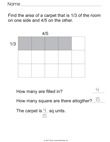Use Tiling To Find Area with Fractional Side Lengths

5.NF.B.5
Interpret multiplication as scaling (resizing), by:
5.NF.B.5.A
Comparing the size of a product to the size of one factor on the basis of the size of the other factor, without performing the indicated multiplication.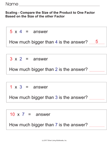Scaling - Compare Product to Factor
5.NF.B.5.B
Explaining why multiplying a given number by a fraction greater than 1 results in a product greater than the given number (recognizing multiplication by whole numbers greater than 1 as a familiar case); explaining why multiplying a given number by a fraction less than 1 results in a product smaller than the given number; and relating the principle of fraction equivalence a/b = (n × a)/(n × b) to the effect of multiplying a/b by 1.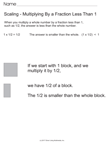Scaling - Multiplying By Fractions
5.NF.B.6
Solve real world problems involving multiplication of fractions and mixed numbers, e.g., by using visual fraction models or equations to represent the problem.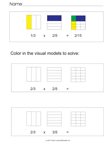Use a Visual Fraction Model to Multiply Fractions

#### Understand decimal notation for fractions, and compare decimal fractions.

5.NF.B.7
Apply and extend previous understandings of division to divide unit fractions by whole numbers and whole numbers by unit fractions.

5.NF.B.7.A
Interpret division of a unit fraction by a non-zero whole number, and compute such quotients. For example, create a story context for (1/3) ÷ 4, and use a visual fraction model to show the quotient. Use the relationship between multiplication and division to explain that (1/3) ÷ 4 = 1/12 because (1/12) × 4 = 1/3.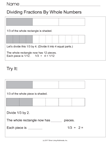Divide Fractions By A Whole Number
5.NF.B.7.B
Interpret division of a whole number by a unit fraction, and compute such quotients. For example, create a story context for 4 ÷ (1/5), and use a visual fraction model to show the quotient. Use the relationship between multiplication and division to explain that 4 ÷ (1/5) = 20 because 20 × (1/5) = 4.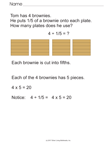Divide a Whole Number By a Fraction (Word Problems)

5.NF.B.7.C
Solve real world problems involving division of unit fractions by non-zero whole numbers and division of whole numbers by unit fractions, e.g., by using visual fraction models and equations to represent the problem. For example, how much chocolate will each person get if 3 people share 1/2 lb of chocolate equally? How many 1/3-cup servings are in 2 cups of raisins?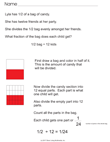Divide A Fraction By a Whole Number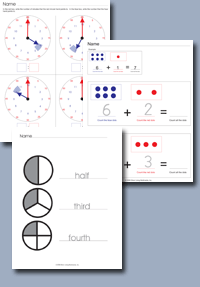Click on any of the standards on the left to create custom worksheets or to print premade materials.